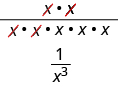## Writing Negative Exponents as Positive Exponents

### Learning Outcomes

• Simplify expressions with negative exponents

The Quotient Property of Exponents has two forms depending on whether the exponent in the numerator or denominator was larger.

### Quotient Property of Exponents

If $a$ is a real number, $a\ne 0$, and $m,n$ are whole numbers, then

${\Large\frac{{a}^{m}}{{a}^{n}}}={a}^{m-n},m>n\text{ and }{\Large\frac{{a}^{m}}{{a}^{n}}}={\Large\frac{1}{{a}^{n-m}}},n>m$

What if we just subtract exponents, regardless of which is larger? Let’s consider ${\Large\frac{{x}^{2}}{{x}^{5}}}$
We subtract the exponent in the denominator from the exponent in the numerator.

${\Large\frac{{x}^{2}}{{x}^{5}}}$   $=$   ${x}^{2 - 5}$   $=$   ${x}^{-3}$

We can also simplify ${\Large\frac{{x}^{2}}{{x}^{5}}}$ by dividing out common factors:This implies that ${x}^{-3}={\Large\frac{1}{{x}^{3}}}$ and it leads us to the definition of a negative exponent.

### Negative Exponent

If $n$ is a positive integer and $a\ne 0$, then ${a}^{-n}={\Large\frac{1}{{a}^{n}}}$.

The negative exponent tells us to re-write the expression by taking the reciprocal of the base and then changing the sign of the exponent. Any expression that has negative exponents is not considered to be in simplest form. We will use the definition of a negative exponent and other properties of exponents to write an expression with only positive exponents.

### example

Simplify:

1. ${4}^{-2}$
2. ${10}^{-3}$

Solution

 1. ${4}^{-2}$ Use the definition of a negative exponent, ${a}^{-n}={\Large\frac{1}{{a}^{n}}}$. ${\Large\frac{1}{{4}^{2}}}$ Simplify. ${\Large\frac{1}{16}}$
 2. ${10}^{-3}$ Use the definition of a negative exponent, ${a}^{-n}={\Large\frac{1}{{a}^{n}}}$. ${\Large\frac{1}{{10}^{3}}}$ Simplify. ${\Large\frac{1}{1000}}$

### try it

When simplifying any expression with exponents, we must be careful to correctly identify the base that is raised to each exponent.

### example

Simplify:

1. ${\left(-3\right)}^{-2}$
2 ${-3}^{-2}$

### try it

We must be careful to follow the order of operations. In the next example, parts 1 and 2 look similar, but we get different results.

### example

Simplify:

1. $4\cdot {2}^{-1}$
2. ${\left(4\cdot 2\right)}^{-1}$

### try it

When a variable is raised to a negative exponent, we apply the definition the same way we did with numbers.

### example

Simplify: ${x}^{-6}$

### try it

When there is a product and an exponent we have to be careful to apply the exponent to the correct quantity. According to the order of operations, expressions in parentheses are simplified before exponents are applied. We’ll see how this works in the next example.

### example

Simplify:

1. $5{y}^{-1}$
2. ${\left(5y\right)}^{-1}$
3. ${\left(-5y\right)}^{-1}$

### try it

Now that we have defined negative exponents, the Quotient Property of Exponents needs only one form, ${\Large\frac{{a}^{m}}{{a}^{n}}}={a}^{m-n}$, where $a\ne 0$ and m and n are integers.

When the exponent in the denominator is larger than the exponent in the numerator, the exponent of the quotient will be negative. If the result gives us a negative exponent, we will rewrite it by using the definition of negative exponents, ${a}^{-n}={\Large\frac{1}{{a}^{n}}}$.